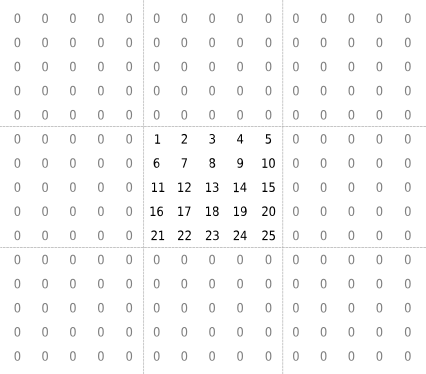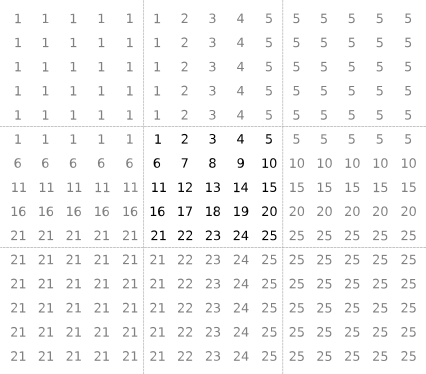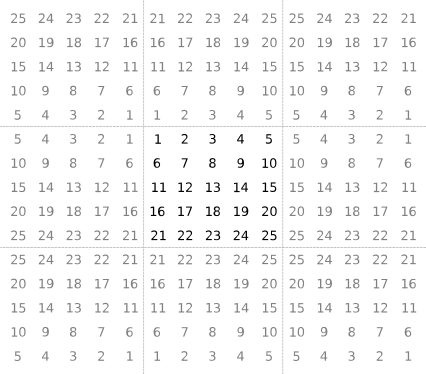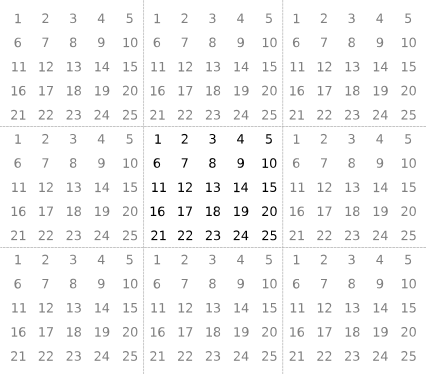# Neighborhood

Control block for Neighborhood Processing Subsystem

## Description

The Neighborhood block serves as a control block for the Neighborhood Processing Subsystem block. The Neighborhood Processing Subsystem block iterates over an input matrix. At each iteration, the inport inside the subsystem receives a small matrix representing a configurable window of elements around one element of the input matrix. Use the Neighborhood block parameters to configure the window dimensions and the size of the output matrix.

## Parameters

expand all

Configure the dimensions of the neighborhood window that the subsystem receives at each iteration. For example, consider this input matrix:

`$\left[\begin{array}{ccccc}1& 2& 3& 4& 5\\ 6& 7& 8& 9& 10\\ 11& 12& 13& 14& 15\\ 16& 17& 18& 19& 20\\ 21& 22& 23& 24& 25\end{array}\right]$`

.

If you set Neighborhood size to `[3 3]`, then on the seventh iteration, the Neighborhood Processing Subsystem block receives this 3-by-3 window that surrounds the seventh element:

`$\left[\begin{array}{ccc}1& 2& 3\\ 6& 7& 8\\ 11& 12& 13\end{array}\right]$`

.

#### Programmatic Use

 Block Parameter: `NeighborhoodSize` Type: array Values: `[3 3]` | `''` Default: `[3 3]`

Configure the dimensions of the output matrix.

• `Same` –– The output matrix uses the same dimensions as the input matrix. For elements near the edges, the neighborhood extends beyond the input matrix. Use the Padding option parameter to configure how to pad elements outside the input matrix.

• `Full` –– The output matrix is larger than the input matrix. It includes an element for every neighborhood that includes at least one element from the input matrix, even if the neighborhood center is outside the input matrix. Use the Padding option to configure how to pad elements outside the input matrix.

• `Valid` –– The output matrix is smaller than the input matrix. The output matrix includes only the elements whose neighborhoods fall entirely within the input matrix.

#### Programmatic Use

 Block Parameter: `OutputSize` Type: character vector Values: `'Same'` | `'Full'` | `'Valid'` Default: `'Same'`

Configure how to pad values outside the input matrix. When you use the `Full` and `Same` output sizes, some neighborhoods extend beyond the input matrix and use these padded values.

To fully illustrate the logic of each padding option, each example graphic shows the 5-by-5 input matrix padded with 5-by-5 matrices. In practice, not every padded value is necessarily part of a neighborhood, depending on the Neighborhood size and Output size parameters. For example, if you set Neighborhood size to `[3 3]` and Output size to `Same`, the subsystem uses only the innermost layer of padded values.

• `Constant` –– Use a configurable constant value for elements outside the input matrix. For example, this matrix uses a constant value of `0`:.

• `Replicate` –– Extend the values of the edge parameters:.

• `Symmetric` –– Mirror the input matrix:.

• `Circular` –– Repeat the input matrix in a tiled pattern:.

#### Programmatic Use

 Block Parameter: `PaddingOption` Type: character vector Values: `'Constant'` | `'Replicate'` | `'Symmetric'` | `'Circular'` Default: `'Constant'`

#### Dependencies

To enable this parameter, set Output size to `Same` or `Full`.

Configure the value to use for the `Constant` padding option.

#### Dependencies

To enable this parameter, set Padding option to `Constant`.

#### Programmatic Use

 Block Parameter: `PaddingConstant` Type: scalar Values: `0` | `''` Default: `0`

## Version History

Introduced in R2022b